## 1. Introduction

MSclassifR is a R package dedicated for Automated classification of mass-spectra with machine learning methods.It was developed with the aim of identifying very similar species or phenotypes of bacteria from mass spectra obtained by Matrix Assisted Laser Desorption Ionisation - Time Of Flight Mass Spectrometry (MALDI-TOF MS). However, the different functions of this package can also be used to classify other categories associated to mass spectra; or from mass spectra obtained with other mass spectrometry techniques. It includes easy-to-use functions for pre-processing mass spectra, functions to determine discriminant mass-over-charge values (m/z) from a library of mass spectra corresponding to different categories, and functions to predict the category (species, phenotypes, etc.) associated to a mass spectrum from a list of selected mass-over-charge values.

In this vignette, we search to discriminate two species of the genus Klebsiella by MALDI-TOF mass spectrometry with a data set of 18 mass spectra (Lasch et al. (2016)) using MSclassifR package. The raw data were downloaded from this link (Lasch et al. (2016)).

## 2. Install packages

The installation of the MSclassifR package requires the installation of packages from Bioconductor, so you might have to install the latest version of the BiocManager package. The MSclassifR package imports the other necessary packages from the CRAN. It is recommended to have the latest version of R.

• Install MSclassifR using the following code:
## install BiocManager if not installed
if (!require("BiocManager", quietly = TRUE))
install.packages("BiocManager")

## Install the mixOmics and multtest packages from Bioconductor
BiocManager::install(c("multtest","mixOmics"))

## Install MSclassifR package
install.packages("MSclassifR")
• Check after install the MSclassifR package:
## For signal processing and machine learning
require(MSclassifR)
## Le chargement a nécessité le package : MSclassifR
## Warning: le package 'MSclassifR' a été compilé avec la version R 4.1.3
• To continue this vignette, we need the MALDIquantForeign, MALDIquant and caret packages from the CRAN. The installation is possible with the command install.packages().
## For additional signal processingt functions

### install MALDIquantForeign and MALDIquant if not installed:
#install.packages("MALDIquantForeign")
#install.packages("MALDIquant")
require(MALDIquantForeign)
## Le chargement a nécessité le package : MALDIquantForeign
## Le chargement a nécessité le package : MALDIquant
##
## This is MALDIquant version 1.21
## Quantitative Analysis of Mass Spectrometry Data
##  See '?MALDIquant' for more information about this package.
require(MALDIquant)

## For additional machine learning functions

### install caret if not installed:
#install.packages("caret")
require(caret)
## Le chargement a nécessité le package : caret
## Warning: le package 'caret' a été compilé avec la version R 4.1.3
## Le chargement a nécessité le package : ggplot2
## Le chargement a nécessité le package : lattice

## 3. Import spectra and metadata

For this vignette, we will load mass spectra and their respective metadata from a public repository on GitHub.

## url for load the  MS data and metadata
MSdataK <- "https://agodmer.github.io/MSData/Klebsiella/KlebsiellaRKIspectra.RData"

load(url(MSMetadataK))

If you want to use your own data with .flex format from Bruker MALDI-TOF MS, you could use importBrukerFlex from MALDIquantForeign package. Of note, you will have to create your own corresponding metadata as a data frame in this case.

## for data .flex format import
#MyBrukerFlexspectra <- MALDIquantForeign::importBrukerFlex("YourPathway")

## 4. Plotting mass spectra

It can be useful to inspect the spectra visually before starting the analysis. This step allows eliminating poor quality spectra.

## Plotting mass spectra
MSclassifR::PlotSpectra(SpectralData=KlebsiellaRKIspectra[])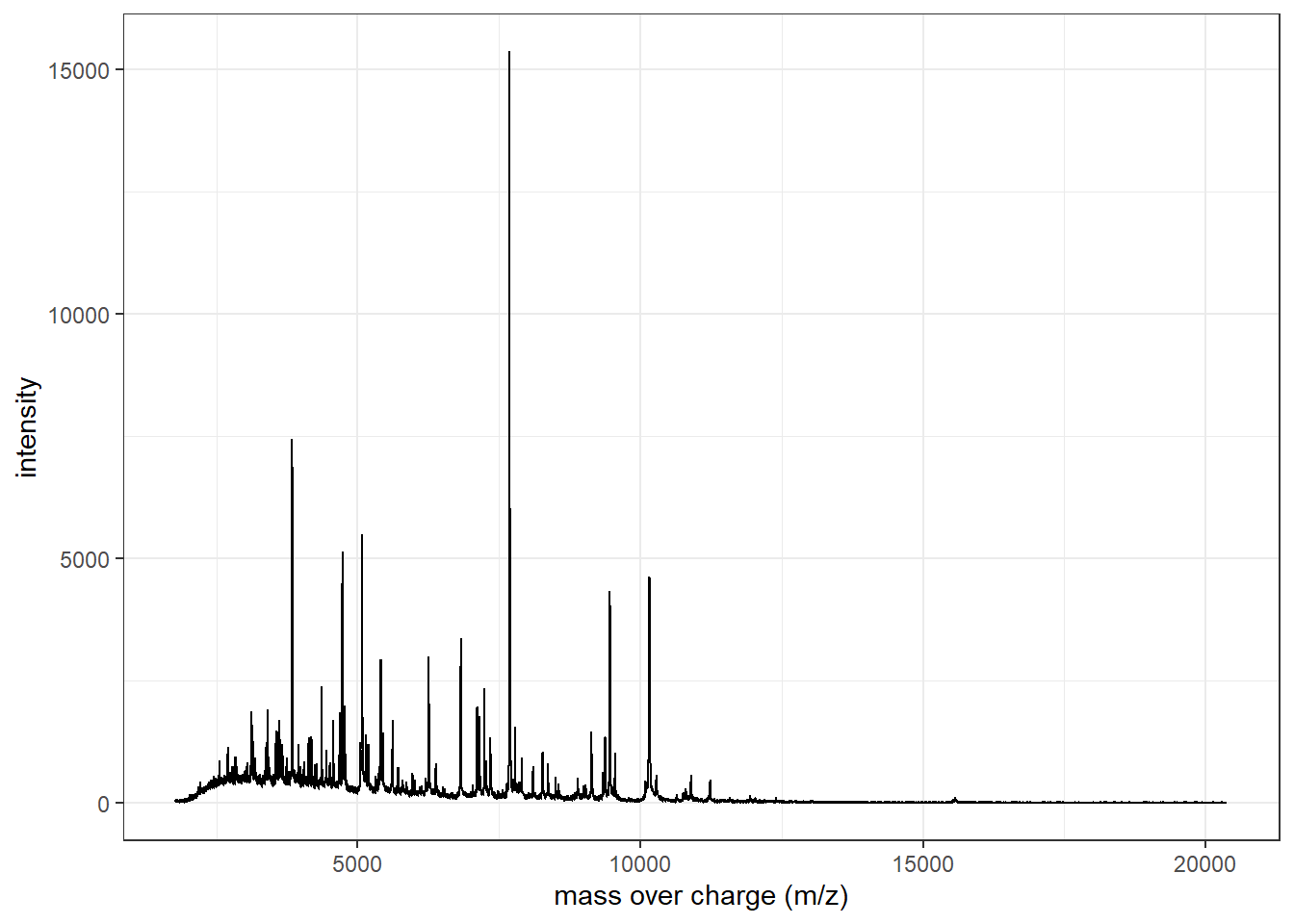## 5. Signal processing and peaks detection

## Signal processing of mass spectra
spectra <- MSclassifR::SignalProcessing(KlebsiellaRKIspectra)
## Peaks detection
peaks <- MSclassifR::PeakDetection(spectra, labels = KlebsiellaRKImetadata$number) You can plot the spectral processing performed and the selected peaks (black crosses). ## Inspect spectra after signal processing MSclassifR::PlotSpectra(SpectralData=spectra[],Peaks=peaks[],col_spec="blue",col_peak="black")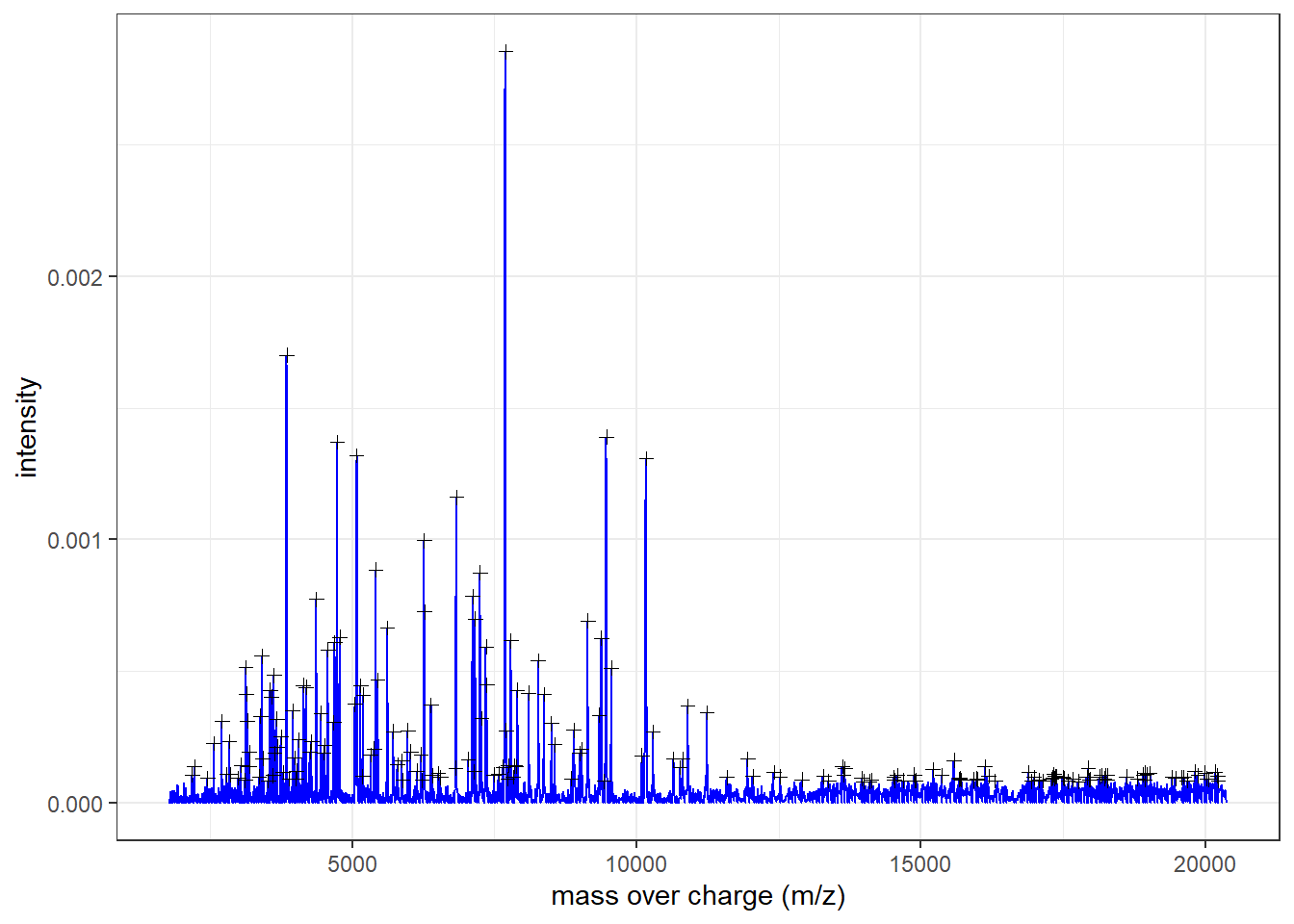At this step, we create an intensity matrix with m/z values in columns and sample names in rows with the intensityMatrix function in MALDIquant package (Gibb and Strimmer (2012)). ## Perfom an Intensity Matrix with spectra data IntMat <- MALDIquant::intensityMatrix(peaks) ## Rows are named according to selected metadata rownames(IntMat) <- paste(KlebsiellaRKImetadata$strains, KlebsiellaRKImetadata$number) ## Remove "NA" in the intensityMatrix IntMat[is.na(IntMat)] <- 0 ## Normalise peaks according to the maximum intensity ## Create basic function norma<-function(x) x/(max(x)) ## Apply this function IntMat <- apply(IntMat,1,norma) ## Transpose Matrix for statistical analysis and named X X <- t(IntMat) Y <- factor(KlebsiellaRKImetadata$species)

The dataset is splitted it into training (70%) and test (30%) datasets using the caretpackage (Kuhn (2008)) and the createDataPartition function for machine learning step.

set.seed(30) # For reproductibility
## Split data to tune the model according train set

## Index creation with p = 0,7
Index_skfold <- caret::createDataPartition(Y, p = 0.7, list=FALSE)

## Train set creation
X_train <- X[Index_skfold,]
Y_train <- Y[Index_skfold]

## Test set creation
X_test <- X[-Index_skfold,]
Y_test <- Y[-Index_skfold]

## Format Y_test as a factor for analysis
Y_testb <- gsub(" ", ".",Y_test)

## 6. Variables selection

The selection of discriminant mass-over-charge values can be performed with several methods proposed in the SelectionVar function of our MSclassifR package. The RFERF method uses the Recursive Feature Elimination (RFE) algorithm coupled with Random Forests (RF); the RFEGlmnet method uses RFE coupled with Logistic regression; the sPLSDA method uses sparse partial least squares discriminant analysis (sPLS-DA) (Rohart F et al. (2017)). The sPLSDA method selects variables from the ones kept in latent components of the model using an automatic choice of the number of components; the VSURF method uses RF with the VSURF function and package (Genuer et al. (2015)).

For this example, we used a Leave-One-Out Cross-Validation (LOOCV) with 5 to 15 variables. The VSURF method performs three steps variables selection : thresholding step, interpretation step and prediction step (Genuer et al. (2015)).

## a. using RFE and Random forest model
set.seed(123) # for  reproducibility
a <- SelectionVar(X_train,
Y_train,
MethodSelection = c("RFERF"),
MethodValidation = c("LOOCV"),
PreProcessing = c("center","scale","nzv","corr"),
Sizes = c(5:15))

## b. using RFE and Random forest model
set.seed(123) # for  reproducibility
b <- SelectionVar(X_train,
Y_train,
MethodSelection = c("RFEGlmnet") ,
MethodValidation = c("LOOCV"),
PreProcessing = c("center","scale","nzv","corr"),
Sizes = c(5:15))

## c. using sPLDA method
set.seed(123) # for  reproducibility
c <- SelectionVar(X_train,
Y_train,
MethodSelection = c("sPLSDA"),
MethodValidation = c("LOOCV"),
PreProcessing = c("scale","nzv"),
Sizes = c(5:15))
## d. using VSURF
set.seed(123) # for  reproducibility
d <- SelectionVar(X_train,
Y_train,
MethodSelection = c("VSURF"))
## Keep selected variables in a list
list_moz <- list("RFERF" = a$sel_moz, "RFEglmnet" = b$sel_moz,
"sPLSDA" = c$sel_moz, "VSURF" = d$sel_moz)
• We can visualize the peaks selected by the RFERF with the red dotted lines.
PlotSpectra(SpectralData=spectra[],Peaks=peaks[],
Peaks2=a$sel_moz,col_spec="blue",col_peak="black")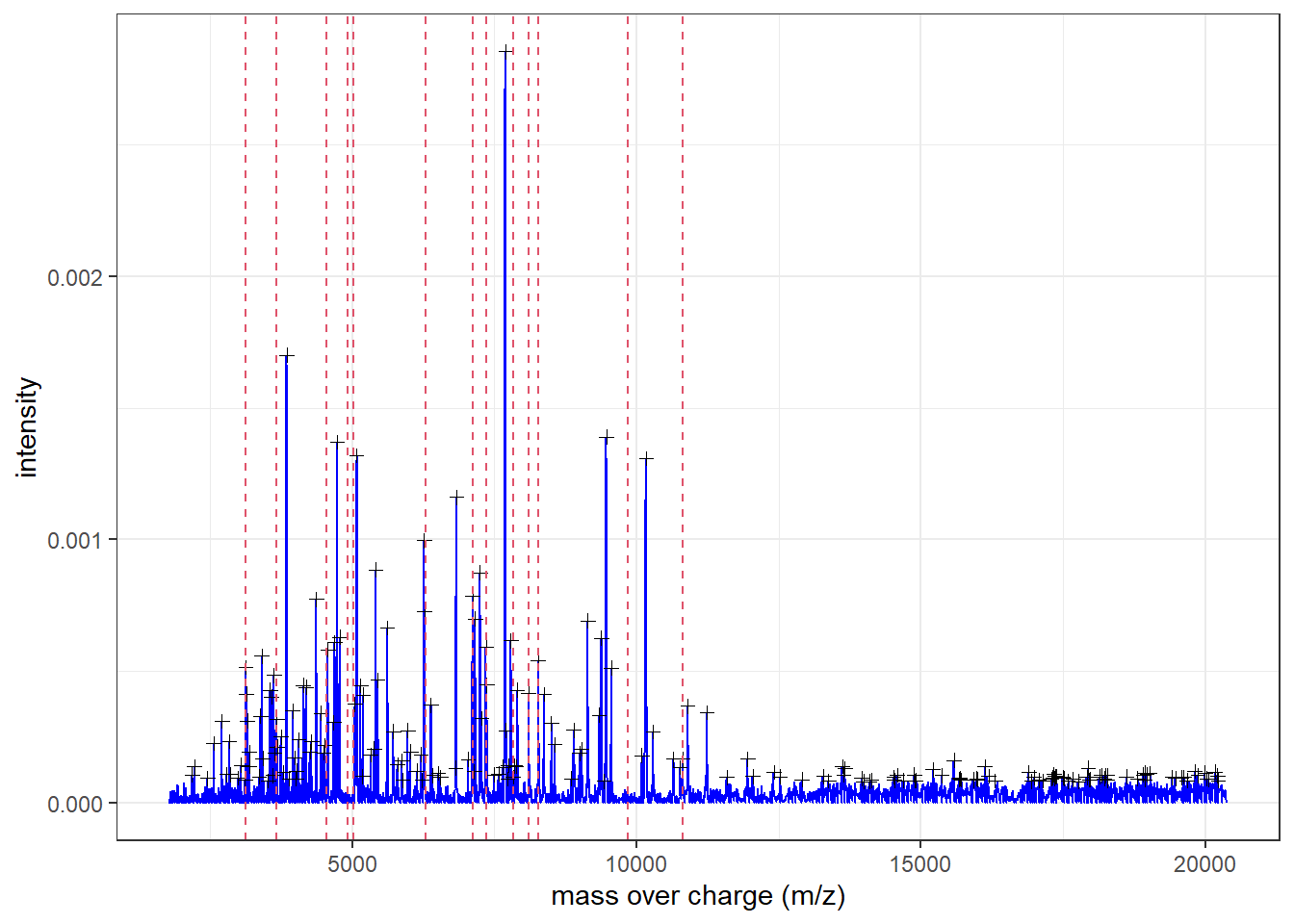• We can visualize the peaks selected by the RFEglmnet method with the red dotted lines. PlotSpectra(SpectralData=spectra[],Peaks=peaks[], Peaks2=b$sel_moz,col_spec="blue",col_peak="black")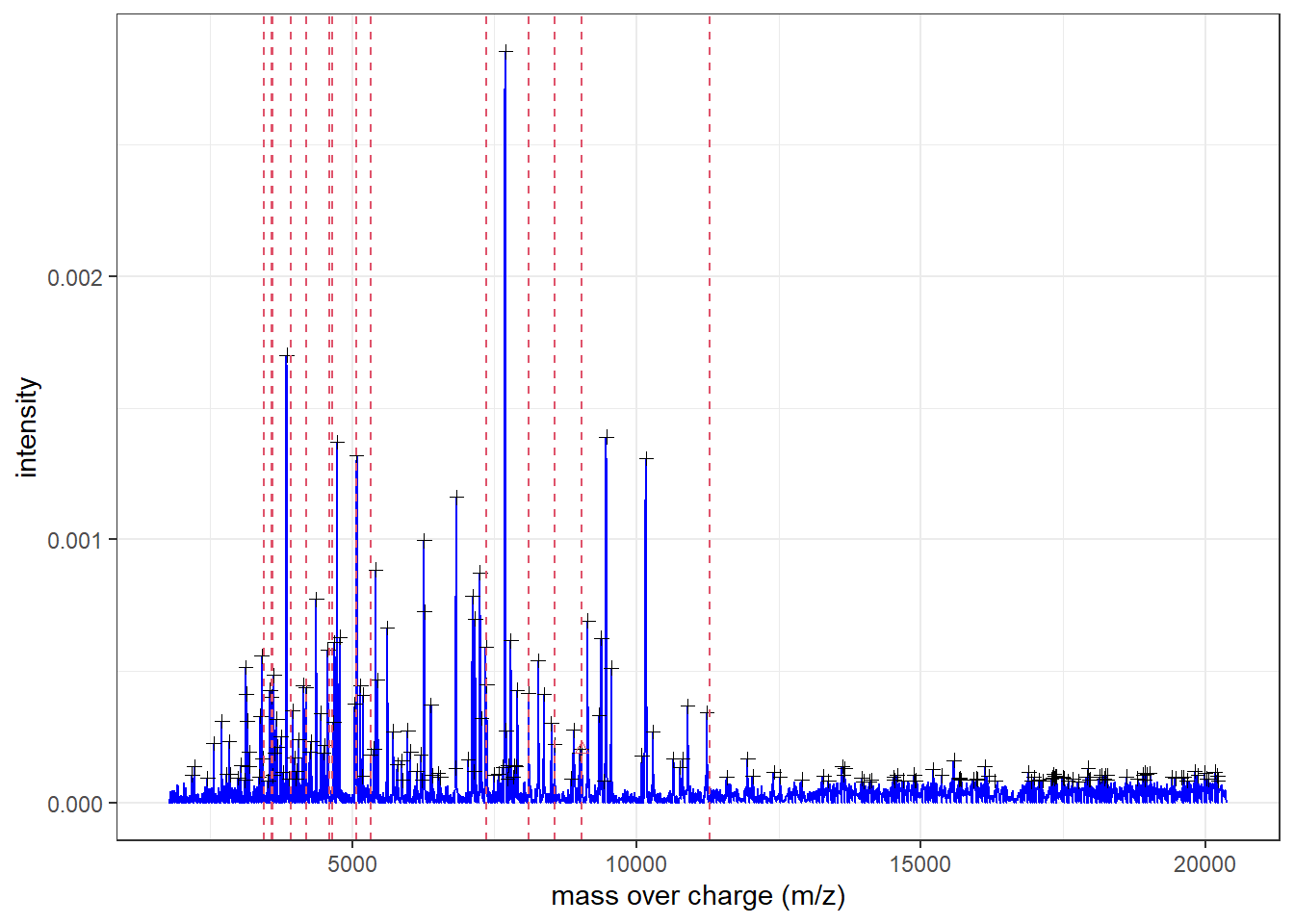• We can visualize the peaks selected by the sPLSDA method with the red dotted lines.
MSclassifR::PlotSpectra(SpectralData=spectra[],Peaks=peaks[],
Peaks2=c$sel_moz,col_spec="blue",col_peak="black")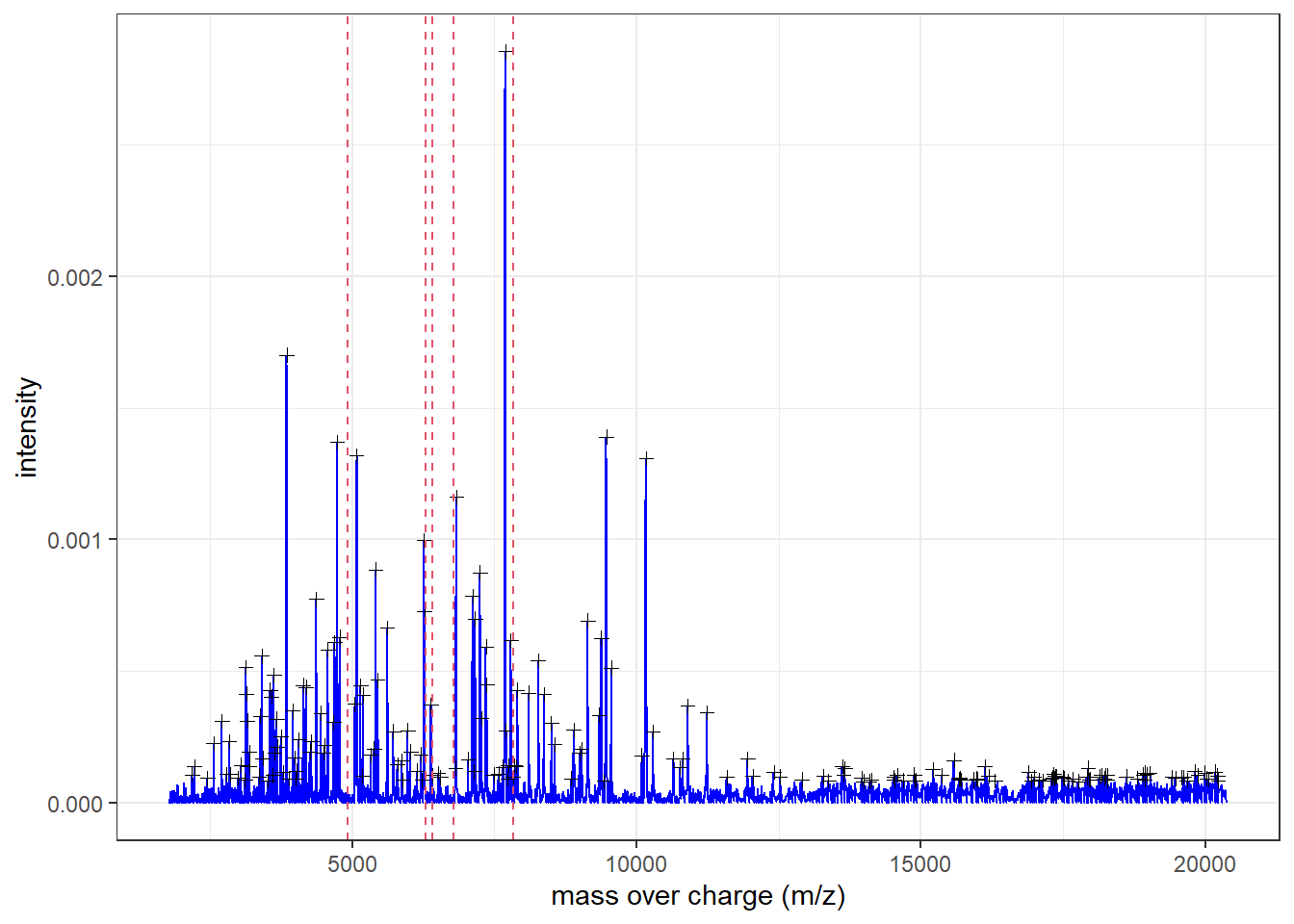• We can visualize the peaks selected by the VSURF method with the red dotted lines. MSclassifR::PlotSpectra(SpectralData=spectra[],Peaks=peaks[], Peaks2=d$sel_moz,col_spec="blue",col_peak="black")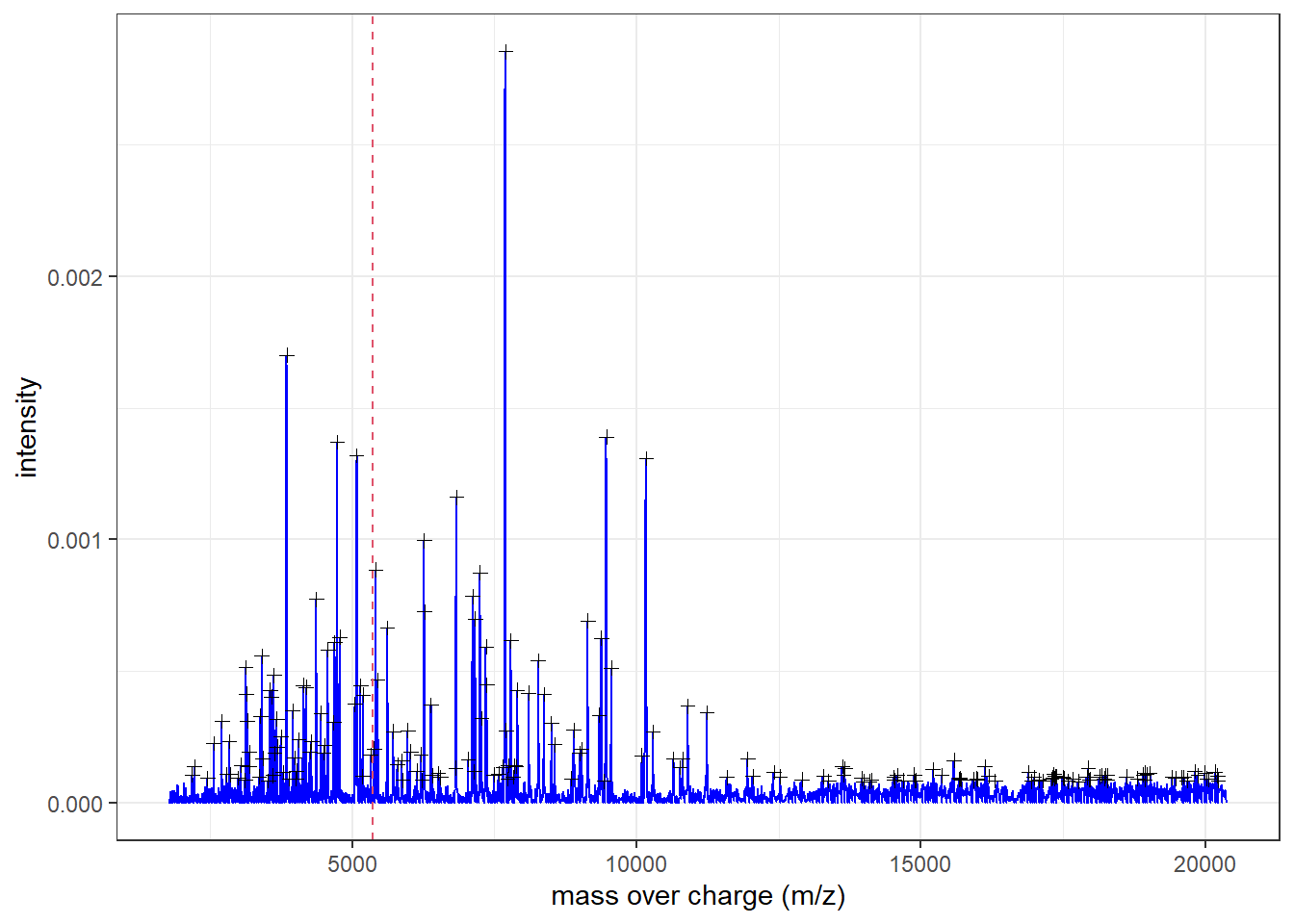• Note: only one masse over charge was selected with VSURF method.This is insufficient to build a prediction model. This selection method will not be included in further analysis.

## 7. Estimation of a multinomial logistic regression

### 7.1 Use the selected variables with RFERF method

With the selected discriminant masses, we can create a prediction model. The LogReg function allows estimating a prediction model with k-fold cross validation in order to predict the category to which a mass spectrum belongs. Two main kinds of models can be estimated: linear or nonlinear (with neural networks (nnet method), random forests (rf method), support vector machines with linear kernel (svm method), or eXtreme Gradient Boosting (xgb method)). Hyperparameters are randomly searched, except for the eXtreme Gradient Boosting where a grid search is performed.

In this part, we will create different prediction models with discriminant mass-over-charge values previously selected ones using the 4 methods described above.

Each prediction models were estimated with a repeated k-fold cross validation (k = 2, and 2 repeats)in the train data set. Next, the accuracies of estimated models are evaluated on the test data set.

## Select variables found with RFERF
sel_moz=a$sel_moz ## linear multinomial regression model_lm=MSclassifR::LogReg(X=X_train, moz=sel_moz, Y=factor(Y_train), number=2, repeats=2) #Estimated model: #model_lm ## nonlinear multinomial regression using neural networks model_nn=MSclassifR::LogReg(X=X_train, moz=sel_moz, Y=factor(Y_train), number=2, repeats=2, kind="nnet") #Estimated model: #model_nn ## nonlinear multinomial regression using random forests model_rf=MSclassifR::LogReg(X=X_train, moz=sel_moz, Y=factor(Y_train), number=2, repeats=2, kind="rf") #Estimated model: #model_rf ## nonlinear multinomial regression using xgboost model_xgb=MSclassifR::LogReg(X=X_train, moz=sel_moz, Y=factor(Y_train), number=2, repeats=2, kind="xgb") #Estimated model: #model_xgb ## nonlinear multinomial regression using svm model_svm=MSclassifR::LogReg(X=X_train, moz=sel_moz, Y=factor(Y_train), number=2, repeats=2, kind="svm") #Estimated model: #model_svm Let’s look at the performances of different prediction models. ## Keep models in a list list_model_A <- list("lm" = model_lm$train_mod,
"nnet" = model_nn$train_mod, "rf" = model_rf$train_mod,
"Xgboost" = model_xgb$train_mod, "svm" = model_svm$train_mod)

## Plot performances of prediction model
model_A <- caret::resamples(list_model_A)
bwplot(model_A)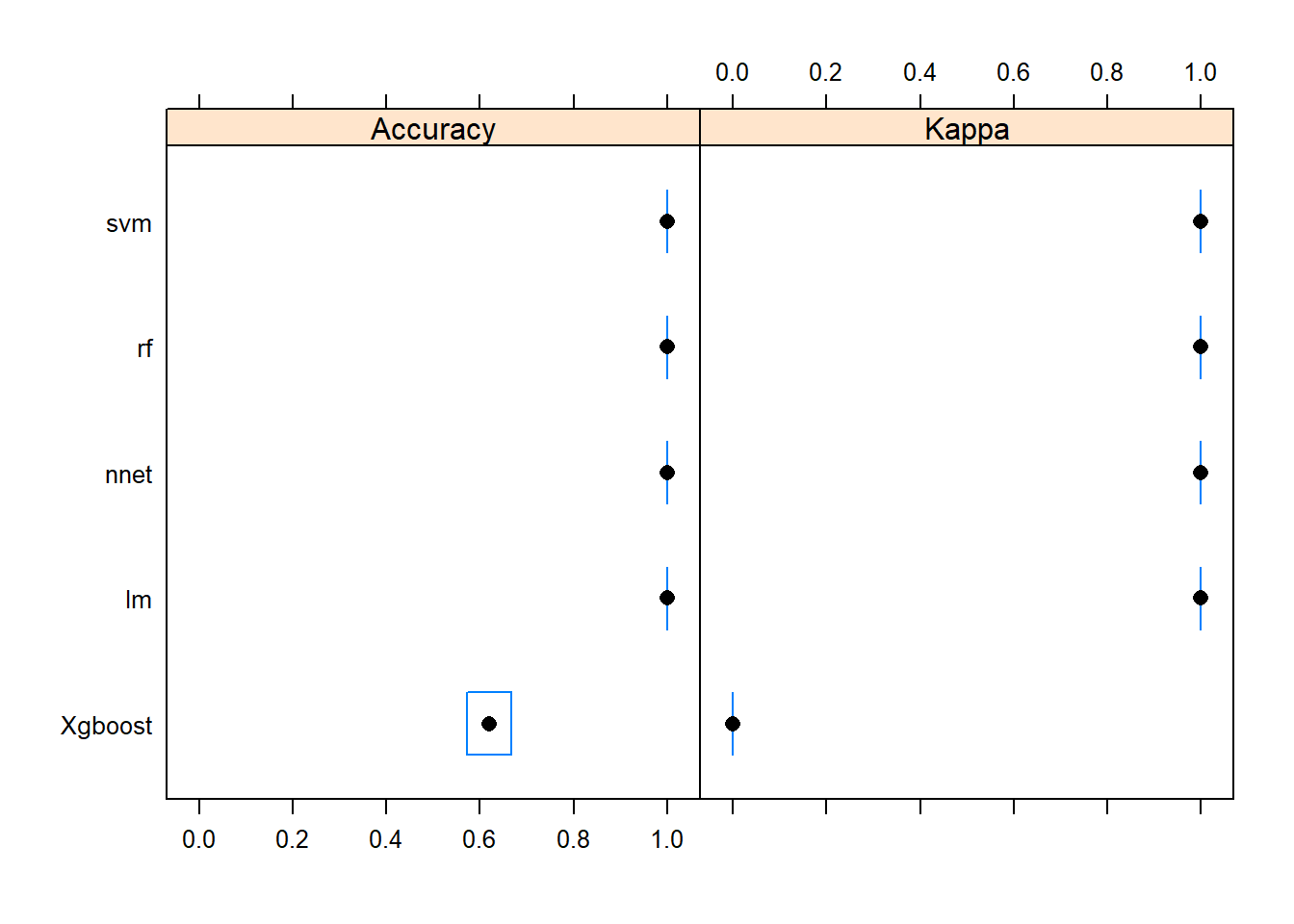## Probabilities of belonging to each category for the mass spectra on test set
prob_cat=MSclassifR::Predict_LogReg(peaks = peaks[-Index_skfold],
model = list_model_A,
moz = sel_moz)
• Estimating accuracies of each method on the test dataset:
## Split table according method used
ResultatsModelA <- split(prob_cat,prob_cat$method) ## Calcul accuracy for each method DF_A <- data.frame(lapply(ResultatsModelA, function(x)(sum(Y_testb == x[,5])/length(Y_testb))), row.names = "RFERF accuracy") t(DF_A) ## RFERF accuracy ## comb_fisher 0.2 ## max_vote 0.4 ## multinom 1.0 ## nnet 1.0 ## rf 1.0 ## svmLinear2 0.8 ## xgbTree 1.0 ### 7.2 Use the selected variables with RFEGlmnet method ## Select variables found with RFGlmnet sel_moz=b$sel_moz

## linear multinomial regression
model_lm=MSclassifR::LogReg(X=X_train, moz=sel_moz, Y=factor(Y_train), number=2, repeats=2)
#Estimated model:
#model_lm

## nonlinear multinomial regression using neural networks
model_nn=MSclassifR::LogReg(X=X_train, moz=sel_moz, Y=factor(Y_train), number=2, repeats=2, kind="nnet")
#Estimated model:
#model_nn

## nonlinear multinomial regression using random forests
model_rf=MSclassifR::LogReg(X=X_train, moz=sel_moz, Y=factor(Y_train), number=2, repeats=2, kind="rf")
#Estimated model:
#model_rf

## nonlinear multinomial regression using xgboost
model_xgb=MSclassifR::LogReg(X=X_train, moz=sel_moz, Y=factor(Y_train), number=2, repeats=2, kind="xgb")
#Estimated model:
#model_xgb

## nonlinear multinomial regression using svm
model_svm=MSclassifR::LogReg(X=X_train, moz=sel_moz, Y=factor(Y_train), number=2, repeats=2, kind="svm")
#Estimated model:
#model_svm
## Keep models in a list
list_model_B <- list("lm" = model_lm$train_mod, "nnet" = model_nn$train_mod,
"rf" = model_rf$train_mod, "Xgboost" = model_xgb$train_mod,
"svm" = model_svm$train_mod) ## Plot performances of prediction model model_B <- caret::resamples(list_model_B) bwplot(model_B)## Probabilities of belonging to each category for the mass spectra on test set prob_cat=MSclassifR::Predict_LogReg(peaks = peaks[-Index_skfold], model = list_model_B, moz = sel_moz) ## Split table according method used ResultatsModelB <- split(prob_cat,prob_cat$method)

## Calcul accuracy for each method
DF_B <- data.frame(lapply(ResultatsModelB, function(x)(sum(Y_testb == x[,5])/length(Y_testb))), row.names = "RFEGlmnet accuracy")

t(DF_B)
##             RFEGlmnet accuracy
## comb_fisher                0.2
## max_vote                   0.4
## multinom                   1.0
## nnet                       1.0
## rf                         1.0
## svmLinear2                 0.2
## xgbTree                    0.8

### 7.3 Use the selected variables with sPLSDA method

## Select variables found with RFGlmnet
sel_moz=c$sel_moz ## linear multinomial regression model_lm=MSclassifR::LogReg(X=X_train, moz=sel_moz, Y=factor(Y_train), number=2, repeats=2) #Estimated model: #model_lm ## nonlinear multinomial regression using neural networks model_nn=MSclassifR::LogReg(X=X_train, moz=sel_moz, Y=factor(Y_train), number=2, repeats=2, kind="nnet") #Estimated model: #model_nn ## nonlinear multinomial regression using random forests model_rf=MSclassifR::LogReg(X=X_train, moz=sel_moz, Y=factor(Y_train), number=2, repeats=2, kind="rf") #Estimated model: #model_rf ## nonlinear multinomial regression using xgboost model_xgb=MSclassifR::LogReg(X=X_train, moz=sel_moz, Y=factor(Y_train), number=2, repeats=2, kind="xgb") #Estimated model: #model_xgb ## nonlinear multinomial regression using svm model_svm=MSclassifR::LogReg(X=X_train, moz=sel_moz, Y=factor(Y_train), number=2, repeats=2, kind="svm") #Estimated model: #model_svm ## Keep models in a list list_model_C <- list("lm" = model_lm$train_mod,
"nnet" = model_nn$train_mod, "rf" = model_rf$train_mod,
"Xgboost" = model_xgb$train_mod, "svm" = model_svm$train_mod)

## Plot performances of prediction model
model_C <- caret::resamples(list_model_C)
bwplot(model_C)## Probabilities of belonging to each category for the mass spectra on test set
prob_cat=MSclassifR::Predict_LogReg(peaks = peaks[-Index_skfold],
model = list_model_C,
moz = sel_moz)
## Split table according method used
ResultatsModelC <- split(prob_cat,prob_cat\$method)

## Calcul accuracy for each method
DF_C <- data.frame(lapply(ResultatsModelC, function(x)(sum(Y_testb == x[,5])/length(Y_testb))), row.names = "sPLSDA accuracy")

t(DF_C)
##             sPLSDA accuracy
## comb_fisher             0.2
## max_vote                0.8
## multinom                0.8
## nnet                    0.8
## rf                      0.8
## svmLinear2              0.4
## xgbTree                 1.0

Prediction models cannot be created with the VSURF method in this example because with the VSURF method because only one variable has been selected. Finally, the accuracies of all the used method in the test dataset can be summarized:

## Summarize results on test set
DFf <- rbind(DF_A, DF_B, DF_C)

## Print results
t(DFf)
##             RFERF accuracy RFEGlmnet accuracy sPLSDA accuracy
## comb_fisher            0.2                0.2             0.2
## max_vote               0.4                0.4             0.8
## multinom               1.0                1.0             0.8
## nnet                   1.0                1.0             0.8
## rf                     1.0                1.0             0.8
## svmLinear2             0.8                0.2             0.4
## xgbTree                1.0                0.8             1.0

## 8. Conclusion

This vignette illustrates how MSclassifR allows estimating many different machine learning-based models to identify two species of the genus Klebsiella from MALDI-TOF mass spectra, and how to use these models to predict the species of a new coming mass spectrum. In the example we used, height models of the 21 models have accuracy equal to 1. For instance, perfect accuracies were obtained on the test data set with variable selection by RFE-RF and RFE-Glmnet coupled with multinom, nnet and rf methods.